Next: 7. Model physics Up: 6. Grids etc Previous: 6.1 Zooming

# 6.2 Slopes and moments

The tracer mass is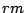(kg) and the air mass is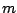(kg) The mixing ratio is then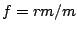(kg tracer/kg air).

A tracer field is defined by 1, 4, or 10 three-dimensional arrays:

array unit description
rm kg toal tracer mass in cell
rxm, rym, rzm kg/(half grid cel) first order moments (slopes)
rxxm, ryym, rzzm
rxym, rxzm, ryzm
kg/(half grid cel)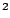second order moments

The slopes and moments are properties of the concentration gradient inside the cell.

Consider a cell volumne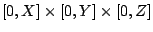. The convention for the length scales is that a half cell has lenght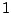, thus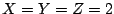. We would like to know the concentration in a pointin the cell. From equations (7) and (9) in [Prather, 1986] we find that the tracer mass mixing ratio (kg tracer/kg air) is given by: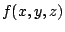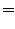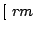(6.1)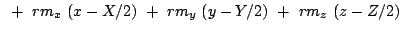(6.2)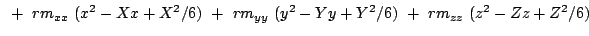(6.3)(6.4)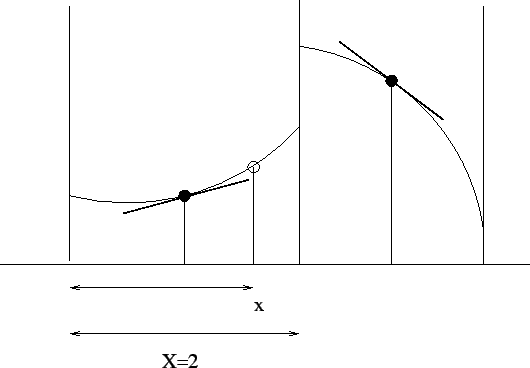Coding of slopes and second moments

The best way to implement slopes/second moments is to have them compiled only if certain preprocessor macro's are defined.

In cy3, at least macro 'slopes' should be defined; in addition, 'secmom' might be defined to.

      ! at least slopes should be defined:
#ifndef slopes
stop 'slopes not defined'
#endif

! second moments as extension of slopes:
#ifdef slopes
rxm = 0.0
#ifdef secmom
rxxm = 0.0
#endif
#endif

! different codes:
#ifdef slopes
#ifndef secmom